# Select and Apply Current Sense Amplifiers Effectively to Better Manage Power

By Art Pini

Contributed By Digi-Key's North American Editors

Power integrity and control is critical for portable, IoT, and automotive devices and systems where line or battery powered electronic devices need to control power distribution by monitoring power supply currents. Current sensing is key to extending battery life, preventing overcurrent conditions, monitoring ground faults, and optimizing control of power sources. The problem is making these measurements accurately in spite of high common mode voltages.

Current sense amplifiers (CSAs) or current shunt monitors are differential amplifier ICs specifically designed for performing this key measurement. Current measurement is based on calculating the voltage drop across series shunt resistors used as current sensors. The selection and placement of these shunts and the associated current sense amplifiers is critical to proper power distribution and efficiency.

This article will describe selection criteria for shunts and current sense amplifiers based on accuracy requirements and cost.

## Resistor current sensing

The simplest technique for measuring current is to insert a small resistor, also called a current shunt, in series with the current to be measured. The voltage across the current sense resistor is measured, and the current is computed using Ohm’s Law based on the known value of the resistor. This method has the advantage of simplicity, low cost, and linearity.

The choice of the current sense resistor must include attention to the resistor’s accuracy, temperature coefficient of resistance (TCR), and power rating. The resistance value determines the voltage drop across it for a given current value. It also determines the power dissipated by the sense resistor. Generally, the sense resistor’s value is a fraction of an ohm. Specialized resistors are available for this application. These resistors use metal elements in the form of plates, foil or film, or deposited thin or thick film hybrid elements.

An example of a metal element surface mount shunt resistor is the Ohmite MCS3264R005FEZR current sensing resistor (Figure 1). This surface mount device (SMD) is a two terminal, 5 milliohm resistor with a 2 watt power rating and a 50 ppm/°C TCR.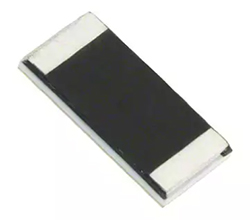Figure 1: The Ohmite MCS3264R005FEZR is a metal element surface mount 5 milliohm shunt resistor. (Image source: Ohmite)

Shunt resistors are also available with a four terminal (Kelvin) configuration. In the Kelvin connection, current is supplied to a pair of source connection terminals. Two additional sense connections (voltage leads) are made immediately adjacent to the shunt resistance. The voltage lead placement avoids the voltage drop associated with source leads or contacts. Since almost no current flows to the measuring instrument, the voltage drop in the sense leads is negligible. The Ohmite FC4TR050FER is an instance of a 50 milliohm, four terminal metal foil current shunt.

It is advisable to keep in mind that the sense resistor’s value will vary with changes in temperature due to the temperature coefficient of resistance. Selecting a resistor with a low TCR, using a resistor with a high power rating, or employing a heatsink are all ways to minimize resistance changes due to temperature effects.

## Current sense amplifiers

A current sense amplifier is a special purpose integrated circuit differential amplifier that is designed to sense the voltage developed across a current shunt and output a voltage proportional to the measured current. The voltage across the current sense resistor is typically in the range of 1 to 100 millivolts, but may ride on the nominal buss voltage potential. The CSA is designed to have a high common mode rejection ratio (CMRR) to eliminate the buss voltage from the output. These devices are designed to handle common mode voltages in excess of their own supply voltage.

The simplified schematic of a current sense amplifier in Figure 2 shows a typical differential amplifier with inverting and non-inverting inputs and a single output.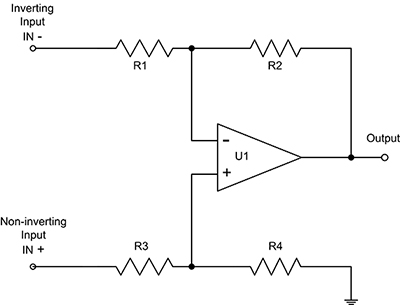Figure 2: The simplified schematic of a typical current sense amplifier. The gain is set by the ratios of resistors R2 to R1 and R4 to R3. (Image source: Digi-Key Electronics)

The resistor values set the gain of the CSA. The structure is symmetrical with R1 = R3 and R2 = R4. The gain is determined by the ratio of R2 to R1 and R4 to R3. In a typical CSA implementation such as the high performance Texas Instruments INA210CIDCKR, R2 and R4 are 1 megohm, and R1 and R3 are 5 kilohms for a gain of 200 volts/volt. The gain accuracy for this version of the amplifier is 0.5%. The rated supply voltage for this IC is from 2.7 to 26 volts, but the maximum common mode input voltage is -3 to 26 volts, regardless of the supply voltage. This is the key distinguishing characteristic of the CSA. The input offset voltage is only 35 microvolts and the CMRR is typically 140 dB.

Depending upon the application, a more economical CSA choice might be the Texas Instruments INA180B3IDBVR. This CSA has an identical common mode input voltage range, and is available with gains of 20, 50, 100, and 200 volts/volt. Gain accuracy is 1% and the CMRR is 100 dB with an input offset voltage of 100 microvolts.

## Current sensing configurations

There are two current sensing topologies; high side and low side sensing. The high side configuration places the sense resistor between the voltage source and the load, while low side sensing places the shunt between the load and ground (Figure 3).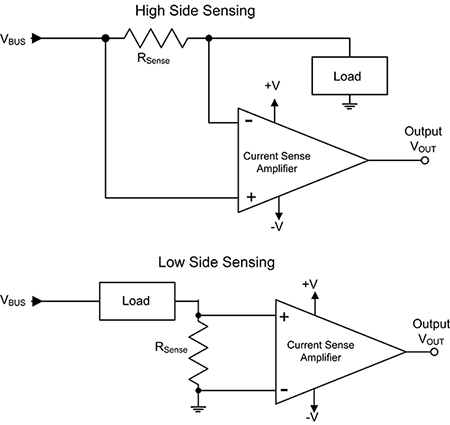Figure 3: High side sensing places the shunt (RSENSE) between the source and the load, while low side sensing places it between the load and ground. (Image source: Digi-Key Electronics)

Low side sensing is ground referenced and has a low input common mode voltage. This makes it easier on the current monitoring amplifier and related circuitry, which usually translates into lower cost.

The disadvantages on the low side connection is that it lifts the load above ground. Current flowing through the shunt resistor raises or lowers the system reference level as current values vary. This can lead to problems in a control loop. Also, short circuits to ground of the voltage bus around the shunt resistor are not detectable in this circuit configuration.

The advantages of the high side topology are that the load and the system reference are fixed at ground independent of the monitored current, and bus shorts to ground are easily detected.

On the down side, there is a common mode voltage close to the bus voltage at the input of the measuring circuit. In addition to stressing the current sense amplifier, in some applications it may also require that the CSA output level be shifted down to near the system reference level.

The issues related to high side sensing have prompted the development of many families of CSAs. The INA180 and INA210 are both new CSAs that can handle common mode voltages of from -3 to 26 volts, independent of their supply voltages. They are intended for applications such as motor control, battery monitoring, and power management, among others. Applications with higher bus voltages can use other CSAs that offer input common mode voltage ranges up to 80 volts. For higher voltages, CSAs require either the use of external components to isolate the amplifier from the common mode voltage, or the use of isolated amplifiers.

## Selecting the sense resistor value

The value of the sense resistor is set to guarantee that the voltage drop across the resistor over the expected bus current range is well above the CSA voltage offset and any additive vertical noise. The power rating of the sense resistor will be determined by the maximum bus current and maximum voltage drop.

Consider as an example a 12 volt bus expected to carry a maximum of 2 amps. If the INA210 CSA is used, the voltage drop across the shunt should be greater than the maximum offset voltage of 35 microvolts.

The common mode rejection ratio is in the range of 105 to 140 dB. Using the lower value (105 dB), the 12 volt bus potential (the common mode voltage), will be attenuated to approximately 67 microvolts. This will appear as an offset voltage at the CSA output, multiplied by the amplifier’s gain. This common mode residue offset is not due to the current being measured, and in this case the residue is not problematic because it is less than 1% of the measured value.

The sense resistor value must be chosen to assure a voltage drop much greater than the offset voltage. For a 2 volt unipolar swing at the output of the INA210, which has a gain of 200, the input should be 10 millivolts. This is significantly greater than the input voltage offset or the common mode residue that was specified. At a nominal maximum current of 2 amps, the sense resistor value should be 5 milliohm. The power rating of the shunt should be at least twice the nominal expected maximum power dissipation of 20 milliwatts. The Ohmite MCS3264R010FEZR described previously would work, given its 2 watt power rating.

Simulating this configuration using the Texas Instruments TINA-TI program we can see the DC and AC transfer characteristics of the circuit (Figure 4). The DC transfer function shows a linear response with a slope of 1 volt/amp. This will produce a 2 volt output for a 2 amp maximum current. The AC response has a bandwidth of 20 kHz.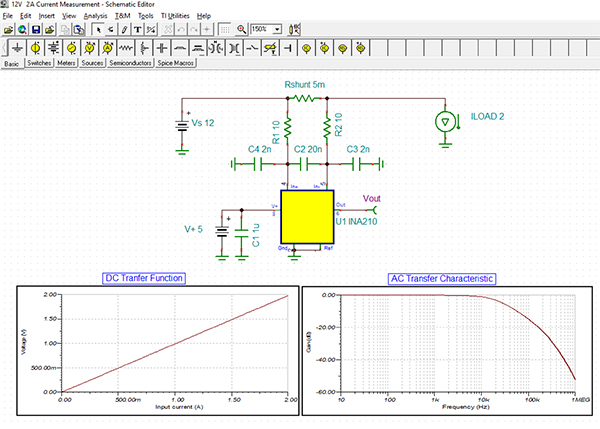Figure 4: A Texas Instruments TINA-TI simulation of the circuit using the 5 milliohm current shunt showing the linear DC transfer function with a 1 volt/amp slope. (Image source: Digi-Key Electronics)

## Conclusion

Current sense amplifiers are specifically designed to measure bus currents based on voltage drops across series shunt resistors. They are particularly suited for high side measurements where high common mode voltages are present. These amplifiers are easy to select, and when used properly, can offer excellent results for the measurement, monitoring, and control of power in electronic systems.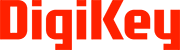Disclaimer: The opinions, beliefs, and viewpoints expressed by the various authors and/or forum participants on this website do not necessarily reflect the opinions, beliefs, and viewpoints of Digi-Key Electronics or official policies of Digi-Key Electronics.

### About this authorArt Pini

Arthur (Art) Pini is a contributing author at Digi-Key Electronics. He has a Bachelor of Electrical Engineering degree from City College of New York and a Master of Electrical Engineering degree from the City University of New York. He has over 50 years experience in electronics and has worked in key engineering and marketing roles at Teledyne LeCroy, Summation, Wavetek, and Nicolet Scientific. He has interests in measurement technology and extensive experience with oscilloscopes, spectrum analyzers, arbitrary waveform generators, digitizers, and power meters.

### About this publisher

Digi-Key's North American Editors